Precalculus : Find the value of any of the six trigonometric functions of quadrantal angles

Example Questions

Example Question #1 : Find The Value Of Any Of The Six Trigonometric Functions Of Quadrantal Angles

Simplify the following expression: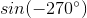Explanation:

Simplify the following expression:Begin by locating the angle on the unit circle. -270 should lie on the same location as 90. We get there by starting at 0 and rotating clockwise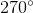So, we know that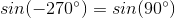And since we know that sin refers to y-values, we know that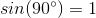So therefore, our answer must be 1

Example Question #31 : Trigonometric Functions

Choose the answer which is equivalent to the following trig expression: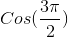Explanation:

Choose the answer which is equivalent to the following trig expression:Begin by finding the location of our given angle. If we start at 0 on the unit circle and go clockwise, every quadrant covers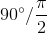Therefore, our given angle will correspond to, which is the bottom half of the y-axis.

Now, because cosine corresponds to the x-value, we know that this expression must be equivalent to 0. If we are on the y-axis, we have no x-value, and therefore, cosine must equal 0

Example Question #1 : Find The Value Of Any Of The Six Trigonometric Functions Of Quadrantal Angles

Which of the following is equivalent to the given expression?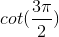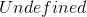Explanation:

Which of the following is equivalent to the given expression?To simplify cotangent expressions, we can think of the expression as tangent and then simply take the reciprocal. So: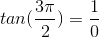, which is undefined.

So,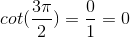Example Question #1 : Find The Value Of Any Of The Six Trigonometric Functions Of Quadrantal Angles

Simplify the following expression: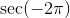Explanation:

Simplify the following expression: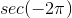I would begin here by recalling that secant is the reciprocal of cosine. Therefore, we can take the cosine of the given angle and then find its reciprocal.

So,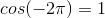(Because cosine refers to x-values and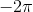lies on the x-axis)

Therefore,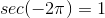Because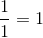.

Example Question #5 : Find The Value Of Any Of The Six Trigonometric Functions Of Quadrantal Angles

Calculate the value of the following trig function: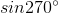Explanation:

Calculate the value of the following trig function:This is a problem which can be instantly solved by a calculator, provided it isn't in radian mode.

However, we are going to run through how to figure this out without a calculator, because knowing how to do it is far more powerful.

Begin by placing the angle in the unit circle.is a multiple of, more specifically, it is three times ninety.

Because we know our angle is three times ninety, we know the angle we are dealing with is one of our four "quadrantal" angles. These are the four that make up our x-y grid.

So,is the angle directly opposite of. This means our x-value must be zero, and our y-value must be.

Now, since we know that sine is basically our y-value, the value ofmust be equal to.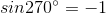All Precalculus Resources